+关注继续查看

# 五、处理分类型特征：编码与哑变量

1. preprocessing.LabelEncoder 标签专用法，将分类转换为分类数值
2. preprocessing.OrdinalEncoder 特征专用法，将分类特征转换为分类数值
3. preprocessing.OneHotEncoder 独热编码，创建哑变量

## （1）preprocessing.LabelEncoder 将标签转换为数值

from sklearn.preprocessing import LabelEncoder
y=data.iloc[:,-1]
y.shape

from sklearn.preprocessing import LabelEncoder
le=LabelEncoder()
le=le.fit(y)
label=le.transform(y)
le.classes_     # 标签中有多少类别
label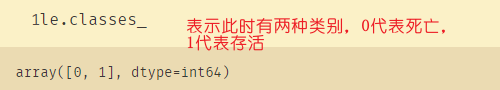le.fit_transform(y)
le.inverse_transform(label) # 0代表死亡，1代表存活

data.loc[:,-1]=label
data.drop(['Survived'],axis=1,inplace=True)
data.rename(columns={-1:'Survived'},inplace=True)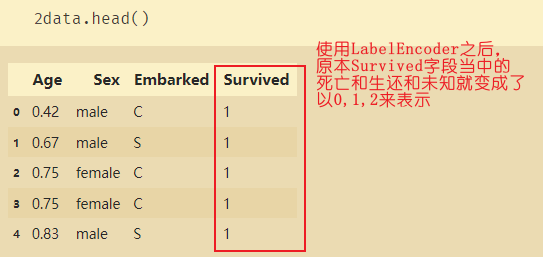## （2）preprocessing.OrdinalEncoder 将特征转换为数值

preprocessing.OrdinalEncoder：特征专用，能够将分类特征转换为分类数值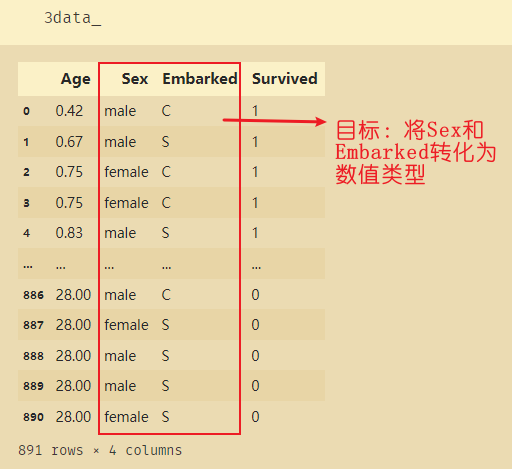from sklearn.preprocessing import OrdinalEncoder
data_=data.copy()
data_

# 取出需要转换的两个字段
OrdinalEncoder().fit(data_.iloc[:,1:-1]).categories_
data_.iloc[:,1:-1]

使用OrdinalEncoder将字符型变成数值
data_.iloc[:,1:-1]=OrdinalEncoder().fit_transform(data_.iloc[:,1:-1])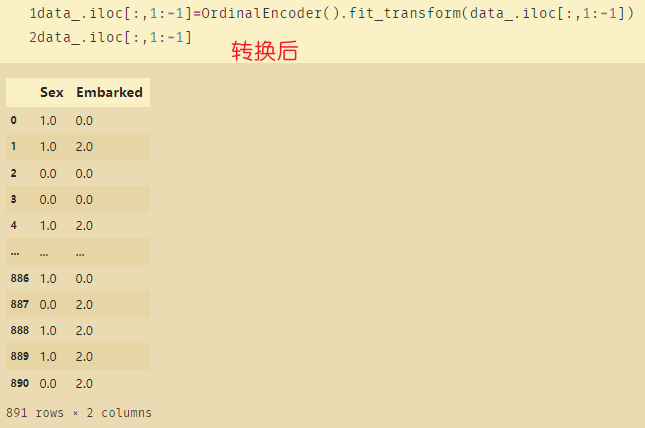## （3）preprocessing.OneHotEncoder 将特征转为稀疏矩阵

OneHotEncoder 独热编码作用：分类编码变量，将每一个类可能取值的特征变换为二进制特征向量，每一类的特征向量只有一个地方是1，其余位置都是0

from sklearn.preprocessing import OneHotEncoder
X=data.iloc[:,1:-1]
X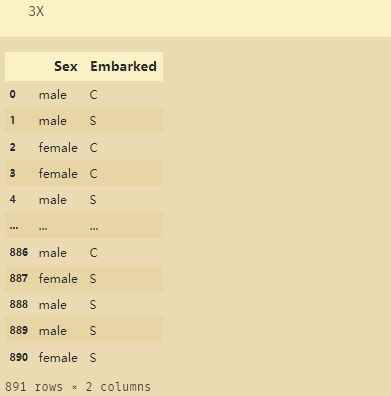enc=OneHotEncoder(categories="auto").fit(X)
enc.transform(X)

result=enc.transform(X).toarray()
result


# 将使用OneHotEncoder后的数据进行还原
pd.DataFrame(enc.inverse_transform(result))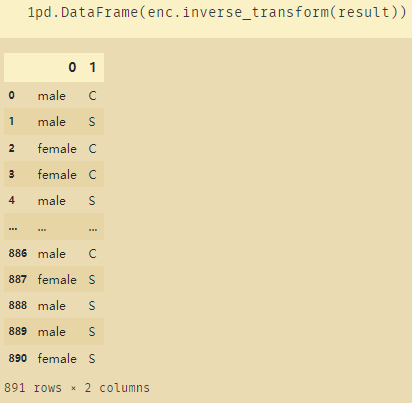enc.get_feature_names()
newdata=pd.concat([data,pd.DataFrame(result)],axis=1)
newdata.drop(['Sex','Embarked'],axis=1,inplace=True)
newdata.columns=['Age','Survived','Female','Male','Embarked_C','Embarked_Q','Embarked_S']
newdata


imp_=SimpleImputer(strategy='most_frequent')
imp_mf=imp_.fit_transform(newdata.iloc[:,1:])
newdata.iloc[:,1:]=imp_mf


# 六、处理连续性特征：二值化与分段

## （1）preprocessing.LabelBinarizer 标签二值化

• 方法一： 求出大于等于1的索引值，令这些索引值对应的数值等于1，然后重新构建列
• 方法二：使用Binarizer(threshold=0.9) 表示大于0.9的数据使用1表示
这里传入的参数需要是二维的，因此需要做维度转换
data_2=data.copy()
from sklearn.preprocessing import Binarizer
x=data_2.iloc[:,0].values.reshape(-1,1)
transformer=Binarizer(threshold=30).fit_transform(x)
transformer

# 将二值化返回newdata
newdata2=pd.concat([newdata,pd.DataFrame(transformer)],axis=1)
# 将二值化后的数据Age_code合并到原本数据当中
newdata2.columns=['Age','Survived','Female','Male','Embarked_C','Embarked_Q','Embarked_S','Age_code']
newdata2.drop(['Age'],axis=1,inplace=True) # 删除原本的Age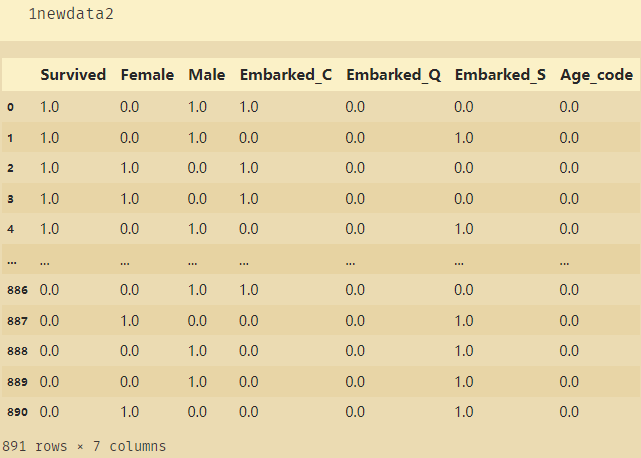# 使用众数填充缺失值
imp_=SimpleImputer(strategy='most_frequent')
imp_mf=imp_.fit_transform(newdata2.iloc[:,-1:])
newdata2.iloc[:,-1:]=imp_mf


## （2）preprocessing.KBinsDiscretizer 连续属性离散化

KBinsDiscretizer 类：这是将连续型变量划分为分类变量的类，能够将连续型变量排序后按顺序分箱后编码。

from sklearn.preprocessing import KBinsDiscretizer
x=data.iloc[:,0].values.reshape(-1,1)
est=KBinsDiscretizer(n_bins=3,encode='ordinal',strategy='uniform')
est.fit_transform(x)

set(est.fit_transform(x).ravel())
est=KBinsDiscretizer(n_bins=3,encode='onehot',strategy='uniform')
est.fit_transform(x).toarray()

newdata3=pd.concat([newdata,pd.DataFrame(est.fit_transform(x).toarray())],axis=1) # 将年龄进行分段
newdata3.columns=['Age','Survived','Female','Male','Embarked_C','Embarked_Q','Embarked_S','Age_1','Age_2','Age_3']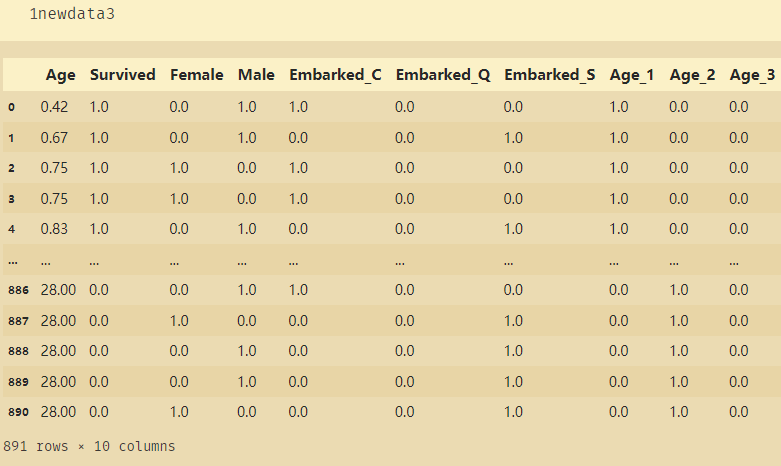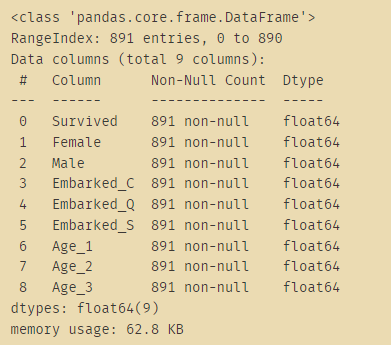|
6天前
|

【机器学习6】数据预处理(三)——处理类别数据(有序数据和标称数据)
【机器学习6】数据预处理(三)——处理类别数据(有序数据和标称数据)
12 0
|
6天前
|

【机器学习4】构建良好的训练数据集——数据预处理(一)处理缺失值及异常值
【机器学习4】构建良好的训练数据集——数据预处理(一)处理缺失值及异常值
11 0
|
4月前
|

90 0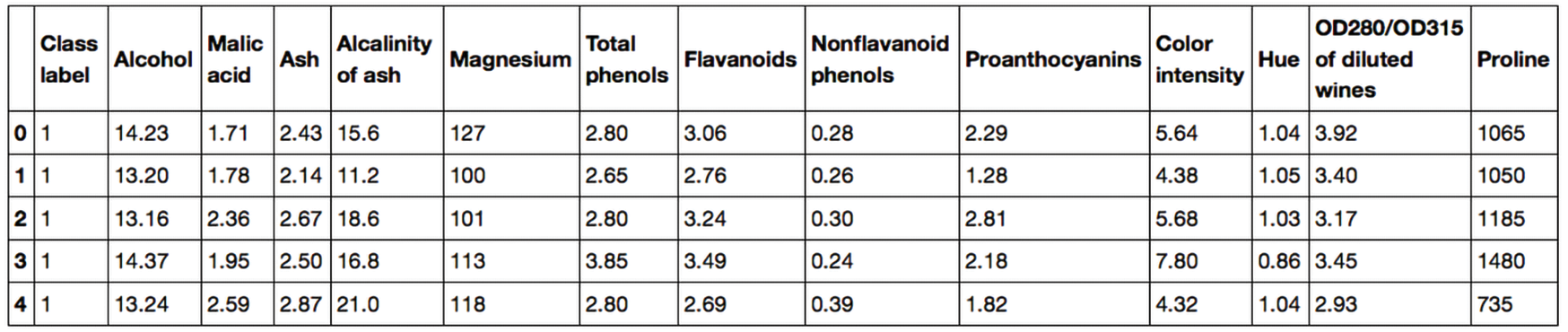|
4月前
|

78 0
|
4月前
|

98 0
|
5月前
|

95 1
|
7月前
|

181 0
|
9月前
|

【机器学习】（27）使用sklearn实现数据预处理
【机器学习】（27）使用sklearn实现数据预处理
75 0
|
9月前
|